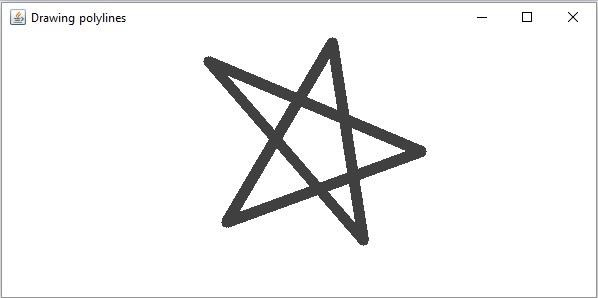# How to draw polylines in OpenCV using Java?

The org.opencv.imgproc package of Java OpenCV library contains a class named Imgproc this class provides various methods to process an input image such as resize(), filter2D, etc.. In addition to these It also provides a set of method to draw geometrical shapes on images.

Among them to draw a polylines you need to invoke the polylines() method of this class. This method accepts the following parameters −

• A Mat object representing the image on which the polygon is to be drawn.

• A-List object holding the objects of the type MatOfPoint.

• A parameter of the type boolean specifying whether the poly-lines are closed.

• A Scalar object representing the color of the polygon.

• An integer representing the thickness of the polygon(default:1).

## Example

import java.util.ArrayList;
import java.util.List;
import org.opencv.core.Core;
import org.opencv.core.Mat;
import org.opencv.core.MatOfPoint;
import org.opencv.core.Point;
import org.opencv.core.Scalar;
import org.opencv.highgui.HighGui;
import org.opencv.imgcodecs.Imgcodecs;
import org.opencv.imgproc.Imgproc;
public class DrawingPolyines {
Mat matrix = null;
public static void main(String args[]) {
//Reading the source image in to a Mat object
//Drawing an polygon
List<MatOfPoint> list = new ArrayList<MatOfPoint>();
new Point(208, 71), new Point(421, 161),
new Point(226, 232), new Point(332, 52),
new Point(363, 250)));
boolean isClosed = true;
Scalar color = new Scalar(64, 64, 64);
int thickness = 10;
Imgproc.polylines (src, list, isClosed, color, thickness);
//Saving and displaying the image
Imgcodecs.imwrite("arrowed_line.jpg", src);
HighGui.imshow("Drawing a polylines", src);
HighGui.waitKey();
}
}

## Output

On executing, the above program generates the following window −